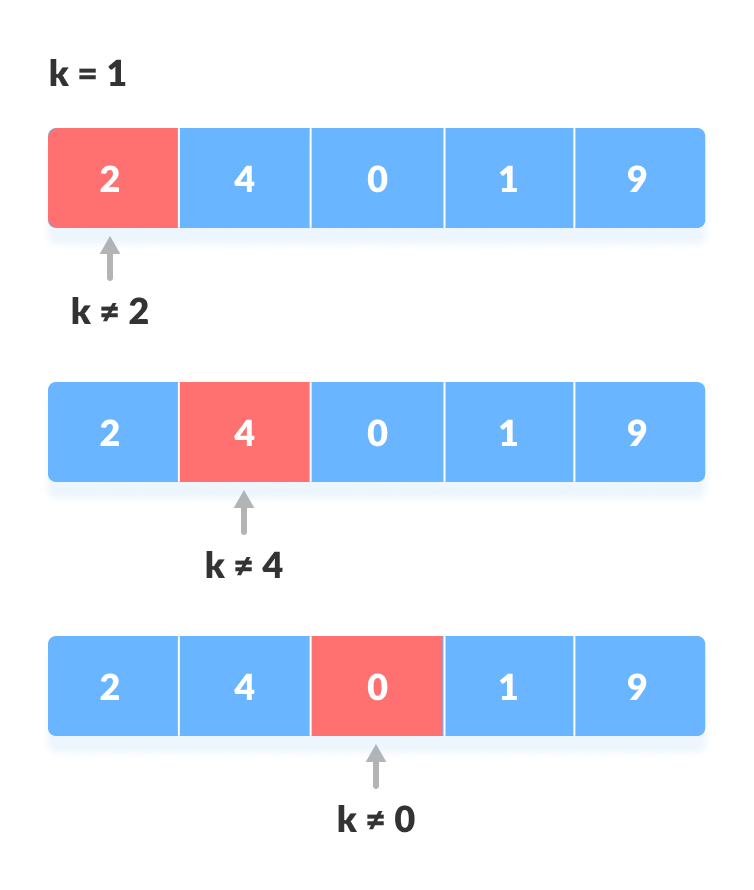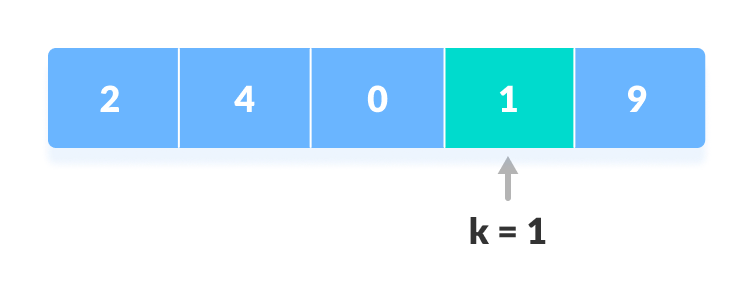Linear Search

# Linear Search

#### In this tutorial, you will learn about linear search. Also, you will find working examples of linear search C, C++, Java and Python.

Linear search is the simplest searching algorithm that searches for an element in a list in sequential order. We start at one end and check every element until the desired element is not found.

## How Linear Search Works?

The following steps are followed to search for an element `k = 1` in the list below.

1. Start from the first element, compare k with each element x.Compare with each element
2. If `x == k`, return the index.Element found

## Linear Search Algorithm

```LinearSearch(array, key)
for each item in the array
if item == value
return its index```

## Python, Java and C/C++ Examples

``````# Linear Search in Python

def linearSearch(array, n, x):

# Going through array sequencially
for i in range(0, n):
if (array[i] == x):
return i
return -1

array = [2, 4, 0, 1, 9]
x = 1
n = len(array)
result = linearSearch(array, n, x)
if(result == -1):
else:
print("Element found at index: ", result)``````
``````// Linear Search in Java

class LinearSearch {
public static int linearSearch(int array[], int x) {
int n = array.length;

// Going through array sequencially
for (int i = 0; i < n; i++) {
if (array[i] == x)
return i;
}
return -1;
}

public static void main(String args[]) {
int array[] = { 2, 4, 0, 1, 9 };
int x = 1;

int result = linearSearch(array, x);

if (result == -1)
else
System.out.print("Element found at index: " + result);
}
}``````
``````// Linear Search in C

#include <stdio.h>

int search(int array[], int n, int x) {

// Going through array sequencially
for (int i = 0; i < n; i++)
if (array[i] == x)
return i;
return -1;
}

int main() {
int array[] = {2, 4, 0, 1, 9};
int x = 1;
int n = sizeof(array) / sizeof(array);

int result = search(array, n, x);

(result == -1) ? printf("Element not found") : printf("Element found at index: %d", result);
}``````
``````// Linear Search in C++

#include <iostream>
using namespace std;

int search(int array[], int n, int x) {

// Going through array sequencially
for (int i = 0; i < n; i++)
if (array[i] == x)
return i;
return -1;
}

int main() {
int array[] = {2, 4, 0, 1, 9};
int x = 1;
int n = sizeof(array) / sizeof(array);

int result = search(array, n, x);

(result == -1) ? cout << "Element not found" : cout << "Element found at index: " << result;
}``````

## Linear Search Complexities

Time Complexity: O(n)

Space Complexity: `O(1)`

## Linear Search Applications

1. For searching operations in smaller arrays (<100 items).